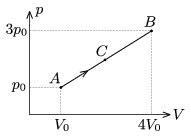Mathematical and Physical Journal
for High Schools
Issued by the MATFUND Foundation
 Already signed up? New to KöMaL?

#Problem P. 4216. (January 2010)

P. 4216. A sample of Helium gas is taken through the process AB, shown in the figure, p0=105 Pa, V0=3 dm3. Determine the pressure and the volume of the gas at state C, until which QAC=3700 J heat is added to the gas.(5 pont)

Deadline expired on February 10, 2010.

Sorry, the solution is available only in Hungarian. Google translation

Megoldás. $\displaystyle 3V_0$ és $\displaystyle \frac{7}{3}p_0$, azaz $\displaystyle 9 \ dm^3$ és $\displaystyle 2,33\cdot 10^5$ Pa.

### Statistics:

 96 students sent a solution. 5 points: 58 students. 4 points: 3 students. 3 points: 1 student. 2 points: 9 students. 1 point: 14 students. 0 point: 5 students. Unfair, not evaluated: 6 solutionss.

Problems in Physics of KöMaL, January 2010